# Get Something for Nothing: Free Power from the Sun!

 Areas of Science Energy & Power Difficulty Time Required Average (6-10 days) Prerequisites None Material Availability Specialty items required. You need to purchase a small solar panel. Cost Average (\$50 - \$100) Safety No issues

## Abstract

Do you like getting something for nothing? Everybody likes getting things for free. How about getting energy and power for free? The Sun sends us free energy every second and all we have to do is collect it. Taking advantage of free energy can reduce our dependence on fossil fuels, which are harmful to our environment. In this science fair project, you will work with a solar panel, which is a collector of free energy, and investigate how varying the angle of the solar panel, and thus the amount of light it absorbs, affects the solar panel's output power.

## Objective

To investigate the effect of the angle of incident light on the output power of a solar panel and determine which angle of incidence produces the most output power.

## Share your story with Science Buddies!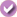Yes, I Did This Project! Please log in (or create a free account) to let us know how things went.

## Credits

Michelle Maranowski, PhD, Science Buddies

General citation information is provided here. Be sure to check the formatting, including capitalization, for the method you are using and update your citation, as needed.

### MLA Style

Science Buddies Staff. "Get Something for Nothing: Free Power from the Sun!" Science Buddies, 12 Jan. 2020, https://www.sciencebuddies.org/science-fair-projects/project-ideas/Energy_p004/energy-power/free-power-from-the-sun. Accessed 21 Jan. 2020.

### APA Style

Science Buddies Staff. (2020, January 12). Get Something for Nothing: Free Power from the Sun! Retrieved from https://www.sciencebuddies.org/science-fair-projects/project-ideas/Energy_p004/energy-power/free-power-from-the-sun

Last edit date: 2020-01-12

## Introduction

Did you know that the core of the Sun is a series of constant nuclear explosions that turn hydrogen into energy? The temperature in the core of the Sun reaches almost 27 million°F, and the Sun produces 3.8x1026 watts (W) of power and 1.4x1031 joules (J) of energy every hour!Figure 1. Shown here is a composite image of multiple solar flares, which are hot eruptions, on the Sun. (JAXA, 2008.)

The Sun provides energy to the Earth in the form of radiated heat and light. The energy that the Earth receives is called insolation. Insolation can be expressed in the units of watts per square meter (W/m2) or kilowatt-hours per square meter (kWh/m2) per day. Of the insolation that arrives at the Earth's upper atmosphere, about half is reflected back into space. The remainder—approximately 50%—of the insolation is absorbed by Earth's oceans and landmass. This means that the Earth is continuously absorbing 89 petawatts! Insolation plays a huge role in many of Earth's functions; for instance, it powers the water cycle and is responsible for almost all of the Earth's renewable energy sources, such as wind and wave energy. There is more than enough free solar energy available to power human energy needs.

Insolation is affected by Earth's atmosphere and the angle of incidence of sunlight on the atmosphere. On a clear day at sea level, when the Sun's rays hit perpendicular to the Earth's surface, we receive 1000 W/m2 of insolation. Because Earth is round, the Sun strikes the surface at different angles, from 0 degrees to 90 degrees. At lower degrees, the insolation travels longer through the atmosphere and becomes scattered and diffuse; whereas at higher degrees, there is less atmosphere to travel through, so more of the Sun's energy hits Earth. The tilt of Earth's axis also affects the amount of insolation reaching the Earth.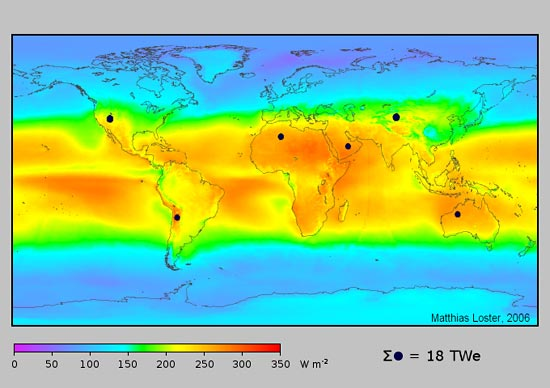The heatmap is color coded by temperature where red areas are the hottest and blue areas are the coolest. The Sun's energy is mostly absorbed near the equator and gradually weakens as you approach either pole.

Figure 2. This is the average annual insolation on the Earth's surface. (Wikipedia, 2008.)

So now you know that the angle of sunlight affects the amount of insolation and power that is absorbed by Earth. But how do we capture and use the insolation? There are many ways of doing this, and this science fair project will allow you to investigate one of those ways: photovoltaic devices.

A photovoltaic device, or solar cell, is a semiconductor device. It is used to convert light into electricity. When a packet of light, called a photon, hits the surface of the solar cell, it is absorbed by the semiconductor. When the photon is absorbed, it creates electrons and holes in the semiconductor. The movement of electrons is electrical current. The amount of electrical current created depends on the amount of insolation, or the number of photons, that hits the surface of the solar cell. Electrical current is proportional to the power that the solar cell delivers. You can learn more about electricity in the Science Buddies Electricity, Magnetism, & Electromagnetism Tutorial.

In this science fair project, you will investigate the output power of a solar panel (a collection of solar cells) as a function of the angle of incoming light (the angle of incidence). Measuring the angle of incoming light is a bit tricky, so you will be changing the angle of incident light by changing the angle of the solar panel. You will model how the Sun interacts with the Earth. Check out this science fair project and get something for nothing!

## Terms and Concepts

• Insolation
• Petawatt
• Angle of incidence
• Photovoltaic
• Solar cell
• Semiconductor
• Electron
• Hole
• Open-circuit voltage
• Short-circuit current
• Scatter plot
• Energy
• Power

### Questions

• What is insolation?
• What affects insolation?
• What is a solar cell?
• How does a solar cell work?
• What are the different materials used to build a solar cell?

## Bibliography

The following websites will give you interesting information on the Sun and solar energy:

The United States Department of Energy's websites have good information and factual links:

This website explains the difference between power and energy:

## News Feed on This Topic, ,
Note: A computerized matching algorithm suggests the above articles. It's not as smart as you are, and it may occasionally give humorous, ridiculous, or even annoying results! Learn more about the News Feed

## Materials and Equipment

• 6 V, 150 mA solar cell with alligator clip leads, Jameco Electronics part #2221456
• Digital multimeter, Jameco Electronics part #2131127
• Desk or table lamp, or a clamp-on reflector lamp (available at a hardware store). Use a high-wattage incandescent bulb (preferably 150 W) or an equivalent CFL or LED bulb.
• Note: because of the spectrum of light emitted, solar cells do not generate as much power from CFL or LED bulbs, but you will still be able to make measurements for purposes of a science project.
• Note: if you do the project outside, you will need to figure out the angle of the sun in the sky, so you can calculate the angle between the solar panel and the sun's rays.
• Scientific calculator
• Lab notebook
• Ruler
.Get Something for Nothing: Free Power from the Sun!

#### https://www.sciencebuddies.org/science-fair-projects/project-ideas/Energy_p004/energy-power/free-power-from-the-sun

PDF date: 2020-01-22

## Experimental Procedure

1. Find an open space that is at least 4 feet long, like a table, to set up your project on. Be sure to have a wall plug close by.
2. Plug in the lamp and place it at one end of the table. Place the solar panel about 2-3 feet away from the lamp. Record how tall the lamp is and how far the lamp is from the solar panel in a data table in your lab notebook.
3. Tilt the solar panel at the lowest angle possible. Calculate the angle of the tilt by looking at Figures 3 and 4 and using Equations 1- 4. Changing the angle of tilt changes the angle of incident light hitting the surface of the solar panel.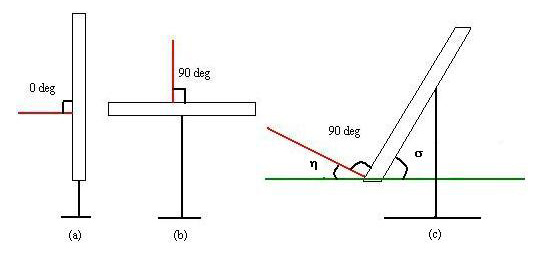Figure 3. This is a schematic of three ways that the solar panel can be placed. (a) The panel at 0 degrees. (b) The panel at 90 degrees, and (c) the panel at an unknown but calculable angle, η.
1. The angle of the solar panel is between a perpendicular line drawn to the surface of the solar panel and a horizontal line. In Figure 3(a), the perpendicular line is at the horizontal line, so the solar panel is at 0 degrees and has no tilt. In Figure 3(b) the perpendicular line is 90 degrees from the horizontal line, so the solar panel is tilted at 90 degrees. Figure 3(c) shows the solar panel tilted at an angle, η. The angle η is calculable using basic trigonometric principles.
2. First calculate the angle σ, shown in Figure 3(c). As shown in Figure 4, use your ruler to measure the height of the solar panel, b, and the length, a.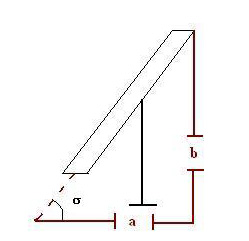Figure 4. Calculating σ is the first step in finding the angle of the solar panel. The value b is the height of the solar panel, while a is the length.
1. As shown in Figure 4, estimate where the solar panel would hit the table surface. That marks the beginning of length a. The height of the solar panel is b. Remember to use the same units for your measurements, either inches or centimeters. Use your scientific calculator and Equation 1, below, to calculate angle σ. Equation 1:
σ = tan-1
(
b
a
)
• σ is in degrees.
• tan-1 is the arctangent.
• b is the height in either inches or centimeters.
• a is the length in either inches or centimeters.
2. Now that you have the angle σ, you can calculate the angle η using Equations 2, 3, and 4. Record the angle in your lab notebook. Note that Equations 2, 3, and 4 are the same equation, just written in different ways. You can see that Equations 2 and 3 are simply rearranged. Equation 4 is made by rearranging the terms from Equation 3, and then plugging in the equation for σ from Equation 1.

Equation 2:

180 degrees = η + 90 degrees + σ

Equation 3:

η = 180 degrees − 90 degrees − σ
Equation 4:
η = 90 degrees − tan-1
(
b
a
)
3. Turn on the lamp. Using the multimeter, measure the open-circuit voltage of the solar panel (if you need help using a multimeter, check out the Science Buddies reference How to Use a Multimeter). Switch to the current setting on the digital multimeter and measure the short-circuit current (you may have to switch the multimeter test leads around to make a current measurement, depending on the kind of multimeter you have). Calculate the maximum power using Equation 5. Record the data in your lab notebook. This number is the maximum power available at this configuration. As soon as you connect a load to the solar panel, such as a resistor or lightbulb, the output power will decrease.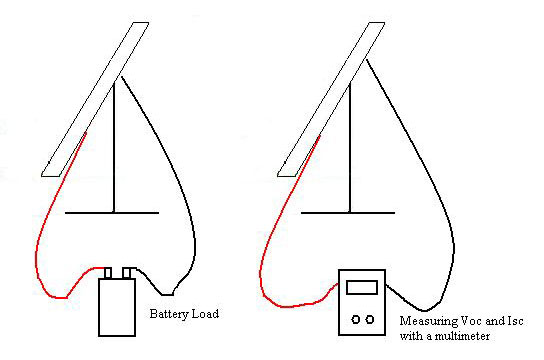Figure 5. Measuring open-circuit voltage and short-circuit current.

Equation 5:
Solar panel maximum output power = Short-circuit current × Open-circuit voltage
Poutput = ISC × VOC

• Poutput is the solar panel maximum output power for the configuration in watts (W).
• ISC is the short-circuit current in amperes (A).
• VOC is the open-circuit voltage in volts (V).

4. Change the angle, or tilt, of the solar panel two more times. Record your data in your lab notebook. Repeat steps 4-9 for each change.
5. Now reset each of the three angles again and repeat the voltage and current measurement two more times for each angle that you chose. You should end up with three sets of data for each of the three angles. Don't forget to record your data in your lab notebook.
6. Plot the collected data. Label the x-axis Panel Angle, η and the y-axis Max Output Power. You can plot the data on a scatter plot or plot the average. How does the power output change with increasing angles? Which angle produced the most output power?

 Solar Panel Angle b a σ η VOC ISC Solar Panel Output Power Angle #1 Trial 1: Trial 1: Trial 2: Trial 2: Trial 3: Trial 3: Average: Average: Angle #2 Trial 1: Trial 1: Trial 2: Trial 2: Trial 3: Trial 3: Average: Average: Angle #3 Trial 1: Trial 1: Trial 2: Trial 2: Trial 3: Trial 3: Average: Average: Lamp position=
.

## If you like this project, you might enjoy exploring these related careers:

### Solar Energy Systems Engineer

Does the idea of harvesting the enormous power of the sun interest you? If you find this exciting, then you should think about installing solar photovoltaic panels on your house to collect free electricity from the sun. But how energy efficient is your home already? Can it get better? How many panels would your house need? What would the system look like? You can get the answers to these questions and more from your local solar energy systems engineer. These engineers help their residential and commercial clients save on their electric bills and reduce their carbon footprint by performing energy audits and picking and designing the right solar energy system for them.

### Solar Photovoltaic Installer

Would you like knowing that what you do every day helps the environment and saves money? Well, that is what solar photovoltaic installers do. They outfit buildings and large solar energy farms with photovoltaic systems that convert free sunlight into clean and renewable energy. This is an exciting, emerging field that will enable you to combine a passion for the environment with mechanical and electrical skills.

### Energy Engineer

How much energy do you think all the houses and buildings in the United States consume? It turns out they eat up 40% of all the energy that the U.S. uses in a year. The figure is high because all those houses and buildings need to be heated, cooled, lit, ventilated, and supplied with heated water and electricity to run all sorts of electrical devices, appliances, and computers. Energy efficiency engineers help reduce the energy that houses and buildings use. This saves families and businesses money, and lowers the emissions of greenhouse gases that contribute to global warming. Read more

## Variations

• Move the lamp either higher or lower to simulate the Sun's movement in the sky. Repeat the science fair project for the lamp's new position.
• Investigate how the output power changes when the area of the solar panel changes. Cover a part of the solar panel with masking tape and see what happens.
• Change the wattage of the lamp and see how the output power varies.

## Share your story with Science Buddies!Yes, I Did This Project! Please log in (or create a free account) to let us know how things went.

The Ask an Expert Forum is intended to be a place where students can go to find answers to science questions that they have been unable to find using other resources. If you have specific questions about your science fair project or science fair, our team of volunteer scientists can help. Our Experts won't do the work for you, but they will make suggestions, offer guidance, and help you troubleshoot.